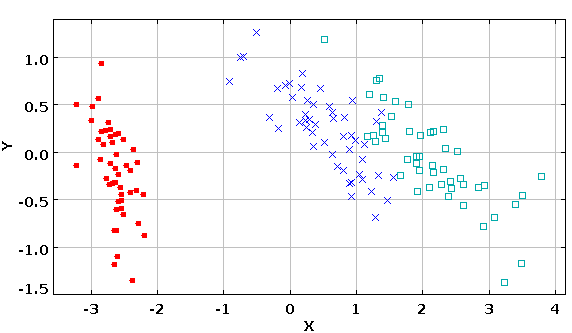# DMelt:Statistics/6 Dimensionality reduction

Limitted access. First login to DataMelt if you are a full DataMelt member. Then login to HandWiki as a user.

# Dimensionality reduction

Dimensionality reduction is a method of transforming complex data in large dimensions into data with lesser dimensions ensuring that it conveys similar information.

Let us consider IRIS dataset . The IRIS data set has 4 numerical attributes. Therefore, it is difficult for humans to visualize such data. Therefore, one can reduce the dimensionality of this dataset down to two. We will use Principal component analysis (PCA) which convert a set of observations of possibly correlated variables into a set of values of linearly uncorrelated variables called principal components. PCA needs the data samples to have a mean of ZERO, so we need a transform to ensue this property as well.

Here is the code that uses the Java packagejsat.datatransform.PCA to perform this transformation:

The output image is shown here:1. Fisher,R.A. "The use of multiple measurements in taxonomic problems", Annual Eugenics, 7, Part II, 179-188 (1936); also in "Contributions to Mathematical Statistics" (John Wiley, NY, 1950).